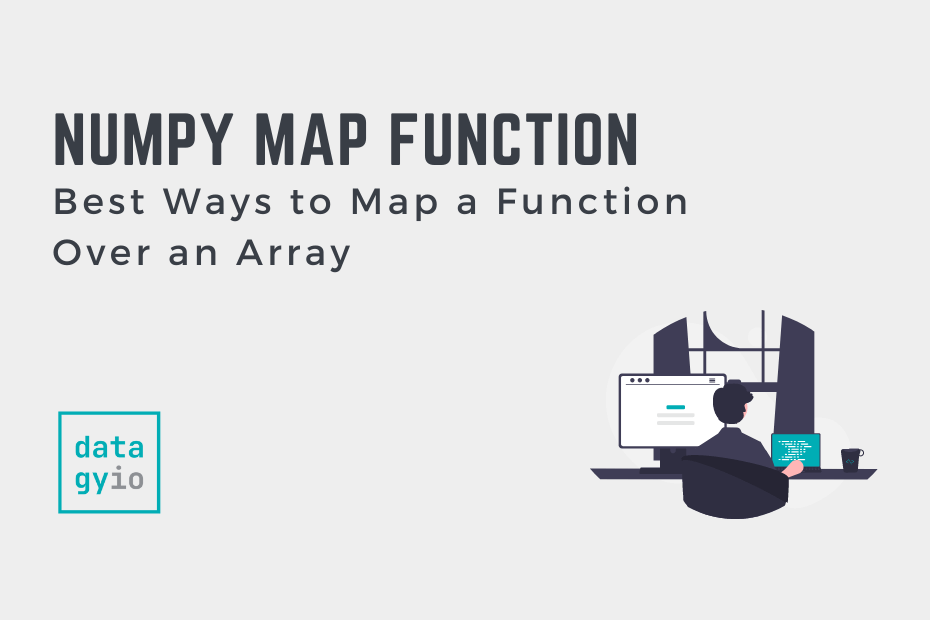# Numpy## NumPy: Best Ways to Map a Function Over an Array

In this tutorial, you’ll learn how to use NumPy to map a function over an array using different methods such as NumPy vectorize. Being able to apply the same function to each element in an array is an important skill.… Read More »NumPy: Best Ways to Map a Function Over an Array## NumPy full: Create Arrays With a Fill Value

NumPy arrays are essential to most data analysis and data science workflows in Python. Because of this, being able to generate arrays is an important skill. In this tutorial, you’ll learn how to use the NumPy full() function to generate… Read More »NumPy full: Create Arrays With a Fill Value## NumPy clip(): Limit Array Values with Min and Max

NumPy arrays are essential to most data analysis and data science workflows. You may want to limit arrays to contain values within a minimum and maximum range – this is what the NumPy clip function accomplishes. In this tutorial, you’ll… Read More »NumPy clip(): Limit Array Values with Min and Max## NumPy cumsum: Calculating Cumulative Sums of NumPy Arrays

The NumPy cumsum function is used to calculate the cumulative sum of elements in a NumPy array across a specified axis. In this tutorial, you’ll learn how to use the NumPy cumsum function to calculate cumulative sums of arrays. The… Read More »NumPy cumsum: Calculating Cumulative Sums of NumPy Arrays## NumPy Histogram: Understanding the np.histogram Function

In this tutorial, you’ll learn how to use the NumPy histogram function to calculate a histogram of a given dataset. A histogram shows the frequency of numerical data in bins of grouped ranges. By using NumPy to calculate histograms, you… Read More »NumPy Histogram: Understanding the np.histogram Function## NumPy arange(): Complete Guide (w/ Examples)

In this guide, you’ll learn how to use the NumPy arange function to create a sequence of numbers. This guide will teach you everything you need to know – including how the function can be customized to meet your needs.… Read More »NumPy arange(): Complete Guide (w/ Examples)## Indexing and Slicing NumPy Arrays: A Complete Guide

This comprehensive guide will teach you all the different ways to index and slice NumPy arrays. NumPy is an essential library for any data analyst or data scientist using Python. Effectively indexing and slicing NumPy arrays can make you a… Read More »Indexing and Slicing NumPy Arrays: A Complete Guide## NumPy argmin(): Get Index of the Min Value in Arrays

In this tutorial, you’ll learn how to master the NumPy argmin() function to find the index position of the minimum value in a NumPy array. The np.argmin() function provides incredible functionality for working with one-dimensional and multi-dimensional arrays. You’ll also… Read More »NumPy argmin(): Get Index of the Min Value in Arrays## Flatten an Array with NumPy flatten

In this tutorial, you’ll learn how to flatten an array with NumPy flatten function, meaning that an array is collapsed to a single dimension. The NumPy flatten function allows you to turn a multi-dimensional array into a single-dimensional array. The… Read More »Flatten an Array with NumPy flatten## NumPy Stack: Join NumPy Arrays Along Different Axes

In this tutorial, you’ll learn how to use the NumPy stack() function to join NumPy arrays along various axes. NumPy is an essential Python library for anyone working with data in Python. The NumPy stack() function allows you to combine… Read More »NumPy Stack: Join NumPy Arrays Along Different Axes## NumPy Squeeze: Removing Dimensionality from NumPy Arrays

In this tutorial, you’ll learn how to use the NumPy squeeze() function. The np.squeeze() function allows you to remove single-dimensional entries from an array’s shape. This allows you to better transform arrays that aren’t shaped in the way that makes… Read More »NumPy Squeeze: Removing Dimensionality from NumPy Arrays## How to Normalize NumPy Arrays

In this tutorial, you’ll learn how normalize NumPy arrays, including multi-dimensional arrays. Normalization is an important skill for any data analyst or data scientist. Normalizing a vector means that its vector magnitude is equal to 1, as a unit vector.… Read More »How to Normalize NumPy Arrays